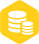# FFT仿真与分析

``````clc;clear all;
fs=50e6;%采样率
N=1024;%采样点数
t=[0:N-1]/fs; %时间序列
f1=3e6;%频点1 3MHZ
f2=4e6;%频点2 4MHZ
s1=sin(2*pi*f1*t);%信号1
s2=sin(2*pi*f2*t);%信号2
mixsign=s1.*s2;%混频
fftsign=fft(mixsign);%求fft
fftabs=abs(fftsign);%取模运算
plot(fftabs);
``````1024点的FFT结果

## 频率分辨率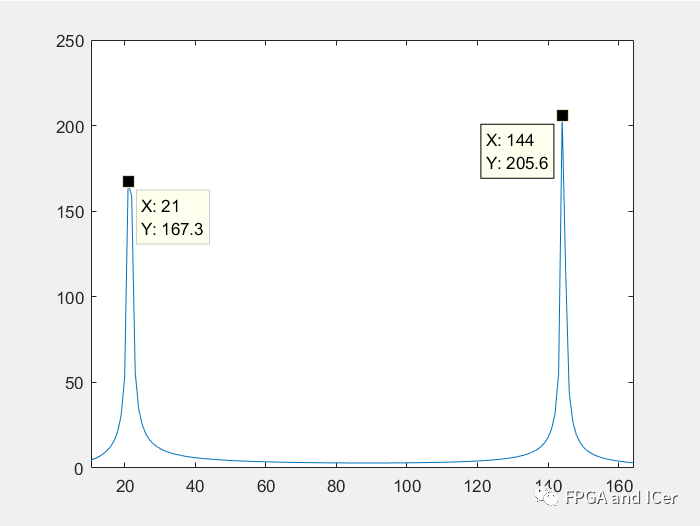FFT结果

# 使用FFT IP进行工程设计

## 实现功率谱逻辑

FFT 的原理是可以通过实部和虚部的数据恢复出周期信号的相位和幅值； 假如 a 是实部数据， b 是虚部数据， a+bj 是复数； 对应的模运算是=sqrt(a^2+b^2)，FFT处理后取模运算中的开更号在FPGA中实现比较麻烦，可以利用自带的cordic IP去处理，这里可以简化一下求FFT处理后的功率谱，也即(a^2+b^2)。

## 添加FFT IP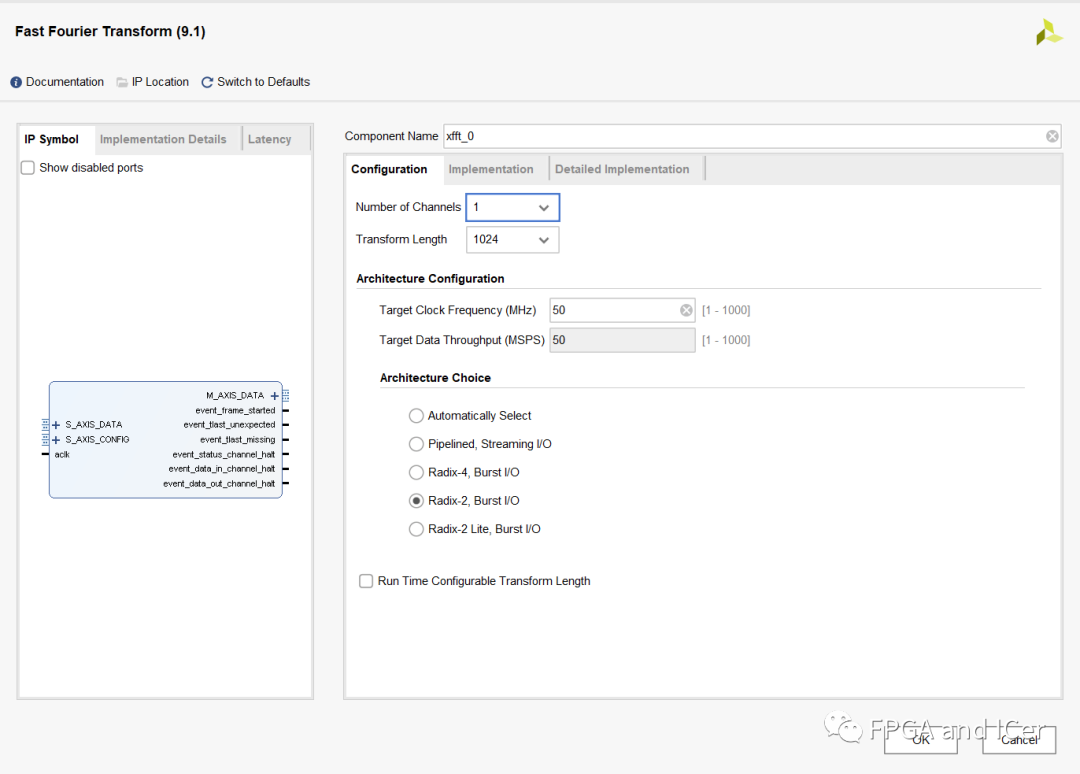FFT IP配置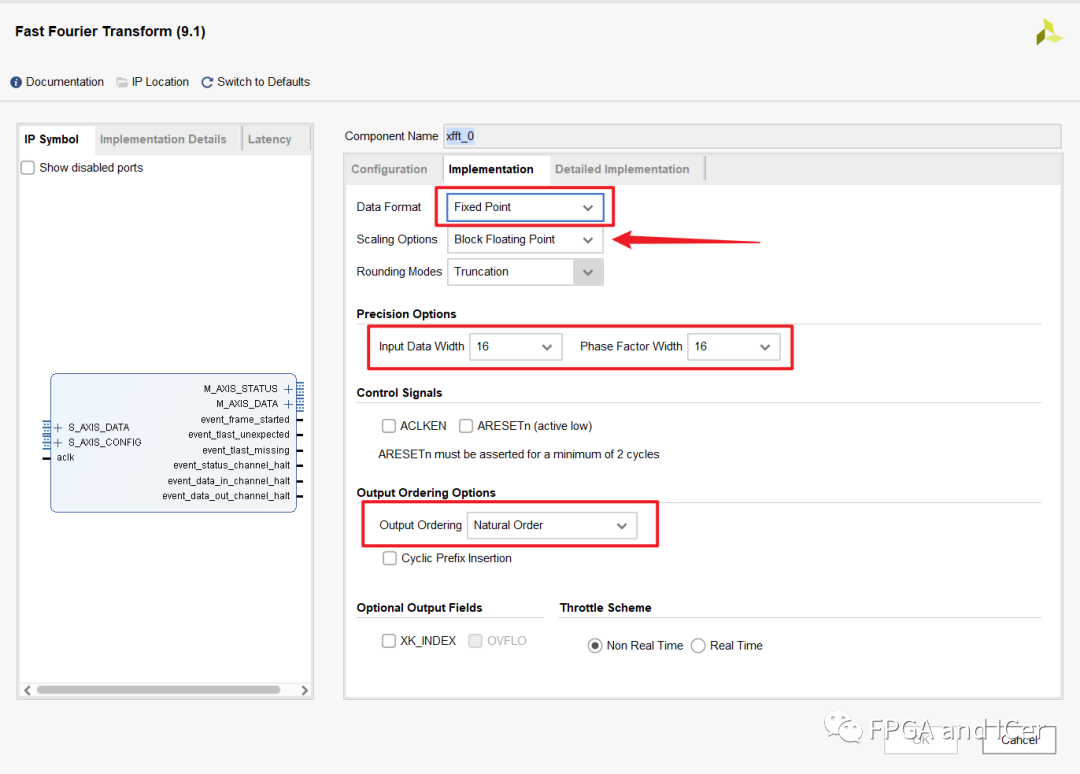FFT ip配置二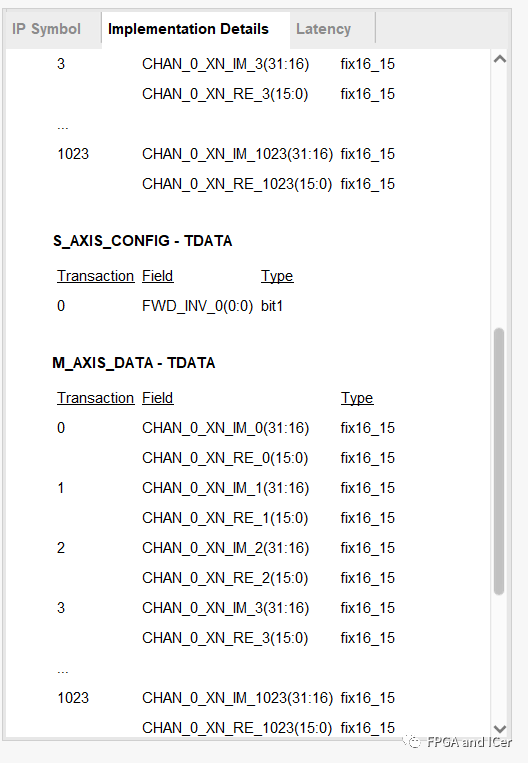FFT侧边栏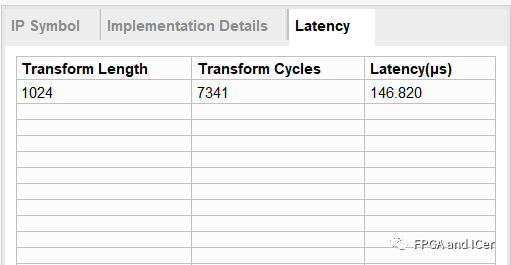## 添加DDS IP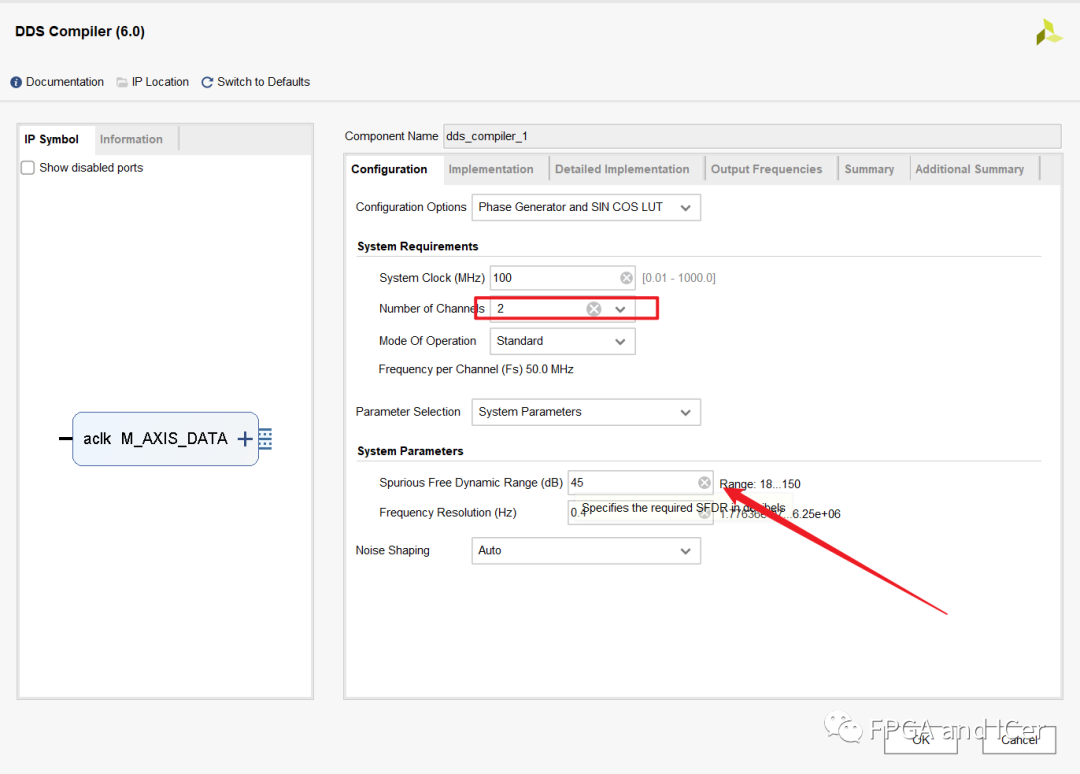DDS ip配置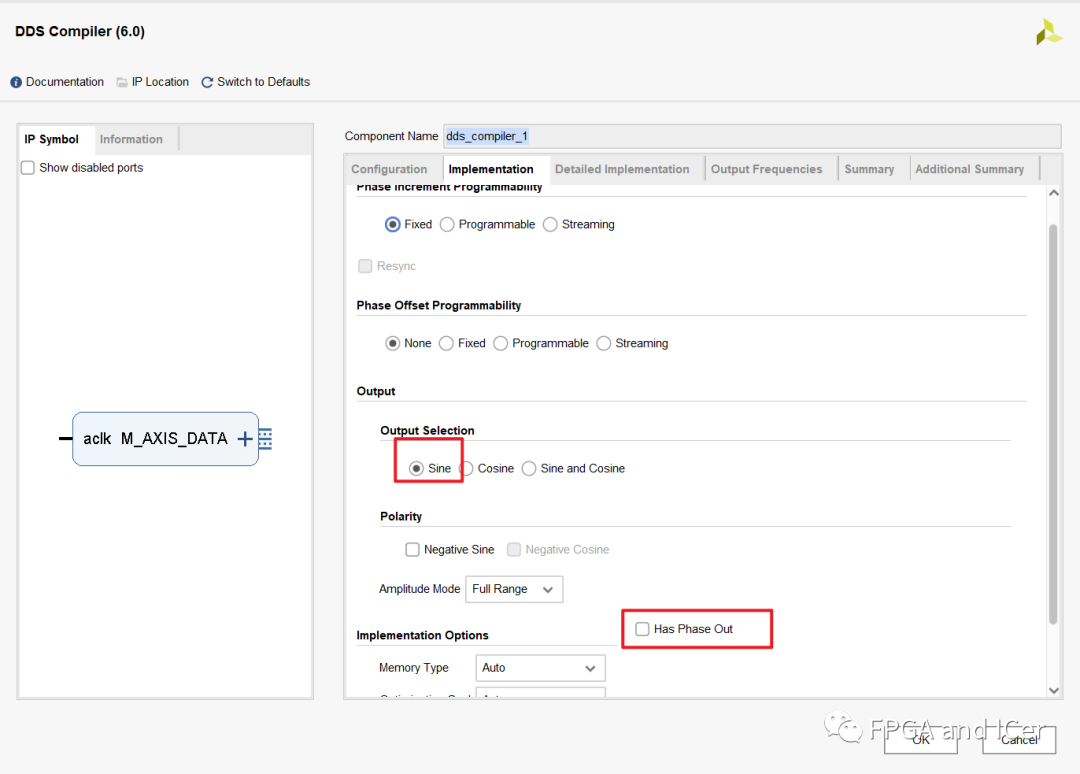DDS的IP核多通道之间是分时复用的，所以在细节实现配置界面最好使能通道ID以供进行区别单个通道的信号波形。 其余可以保持默认。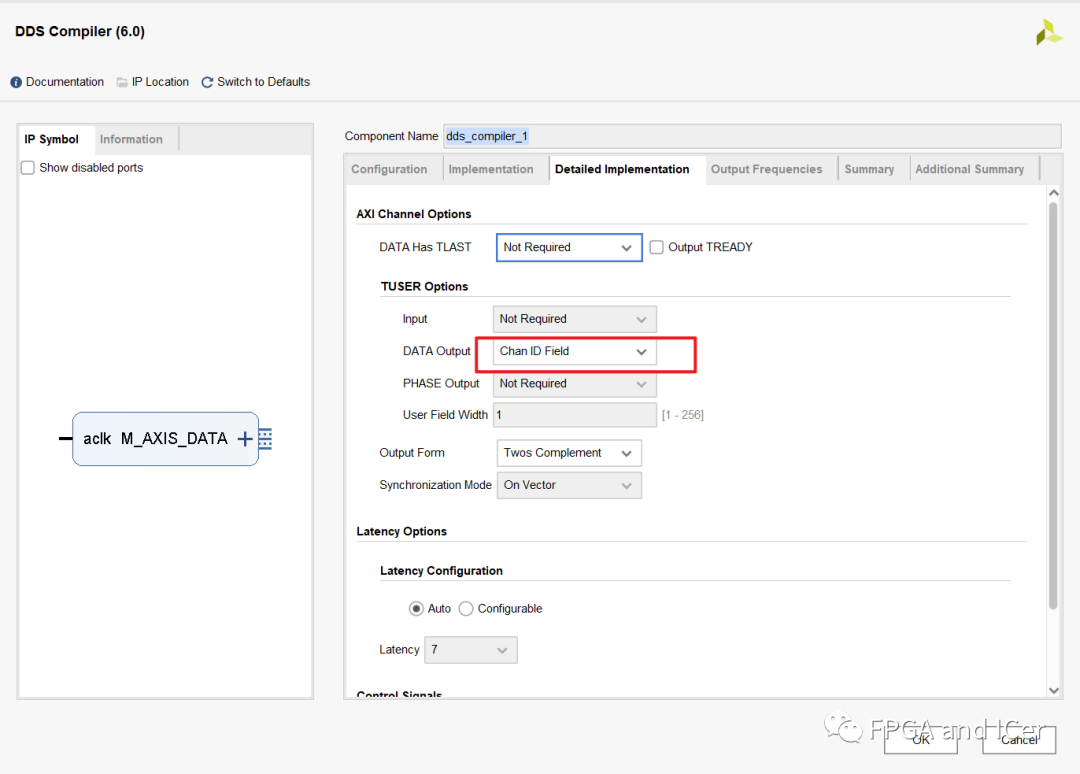## 修改乘法器IP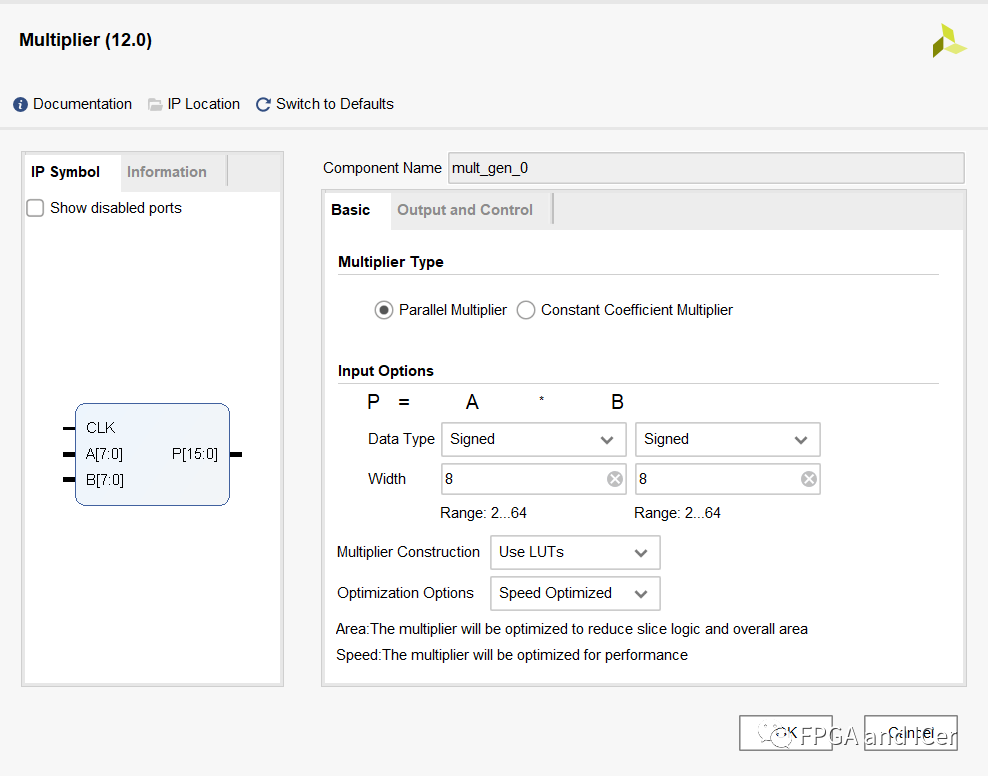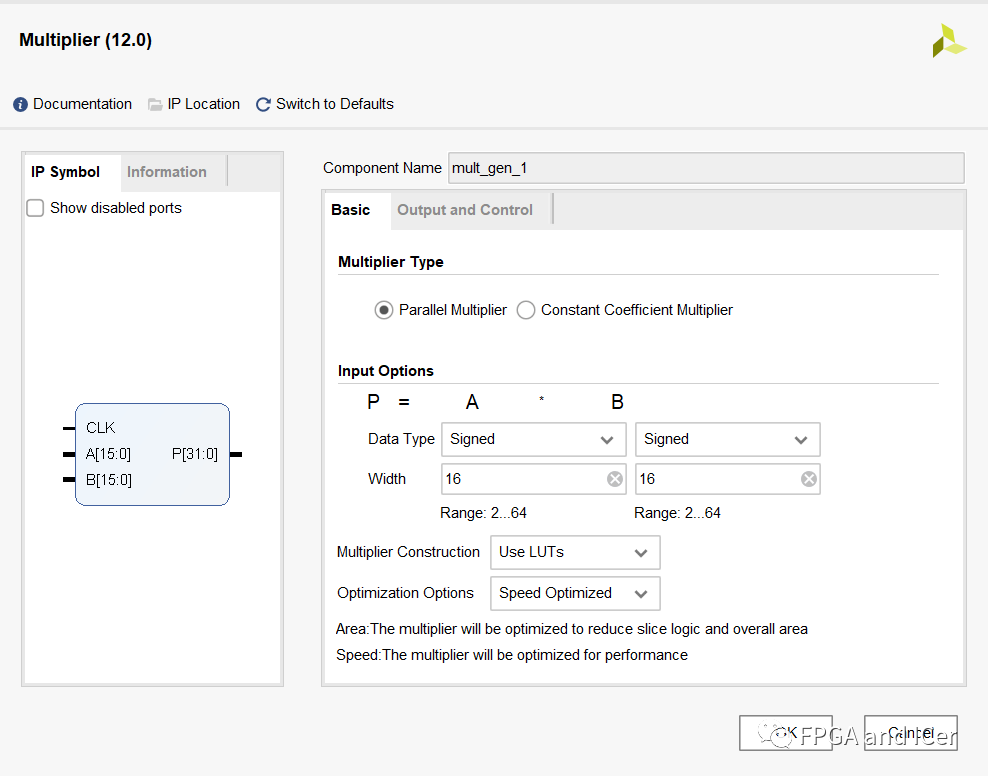## 编写. V文件

```````timescale 1ns / 1ps
module top(
input clk
);

wire          m_axis_data_tvalid_ch3;
wire [7 : 0]  m_axis_data_tdata_ch3;
wire [0 : 0]  m_axis_data_tuser_ch3;

//多通道测试
dds_compiler_1 multi_ch_dds(
.aclk(clk),                                // input wire aclk
.m_axis_data_tvalid(m_axis_data_tvalid_ch3),    // output wire m_axis_data_tvalid
.m_axis_data_tdata(m_axis_data_tdata_ch3),      // output wire [7 : 0] m_axis_data_tdata
.m_axis_data_tuser(m_axis_data_tuser_ch3)
);

reg [7 : 0] data3MHz;
reg [7 : 0] data4MHz;
always @(posedge clk) begin
case(m_axis_data_tuser_ch3)
0:data3MHz<=m_axis_data_tdata_ch3;
1:data4MHz<=m_axis_data_tdata_ch3;
endcase
end

//混频测试
wire [15 : 0]  mixer_singal;
mult_gen_0 mult_mixer (
.CLK(clk),  // input wire CLK
.A(data3MHz),      // input wire [7 : 0] A
.B(data4MHz),      // input wire [7 : 0] B
.P(mixer_singal)      // output wire [15 : 0] P
);

reg div_clk=0;
always @(posedge clk ) begin
div_clk<=!div_clk;
end

wire [31 : 0] after_fft_data;
wire [7 : 0] m_axis_data_tuser;
wire m_axis_data_tvalid;

xfft_0 uut_fft(
.aclk(div_clk),                                                // input wire aclk
.s_axis_config_tdata('d1),                  // input wire [7 : 0] s_axis_config_tdata
.s_axis_config_tvalid(1),                // input wire s_axis_config_tvalid
.s_axis_data_tdata({16'd0,mixer_singal}),                      // input wire [31 : 0] s_axis_data_tdata
.s_axis_data_tvalid(1),                    // input wire s_axis_data_tvalid
.s_axis_data_tlast(0),                      // input wire s_axis_data_tlast
.m_axis_data_tdata(after_fft_data),                      // output wire [31 : 0] m_axis_data_tdata
.m_axis_data_tuser(m_axis_data_tuser),                      // output wire [7 : 0] m_axis_data_tuser
.m_axis_data_tvalid(m_axis_data_tvalid),                    // output wire m_axis_data_tvalid
.m_axis_data_tlast(),                      // output wire m_axis_data_tlast
.m_axis_status_tdata(),                  // output wire [7 : 0] m_axis_status_tdata
.m_axis_status_tvalid(),                // output wire m_axis_status_tvalid
.event_frame_started(),                  // output wire event_frame_started
.event_tlast_unexpected(),            // output wire event_tlast_unexpected
.event_tlast_missing(),                  // output wire event_tlast_missing
.event_status_channel_halt(),      // output wire event_status_channel_halt
.event_data_in_channel_halt(),    // output wire event_data_in_channel_halt
.event_data_out_channel_halt()  // output wire event_data_out_channel_halt
);

wire [31 : 0] fft_re_2;
mult_gen_1 mult_re (
.CLK(div_clk),  // input wire CLK
.A(after_fft_data[15:0]),      // input wire [15 : 0] A
.B(after_fft_data[15:0]),      // input wire [15 : 0] B
.P(fft_re_2)      // output wire [31 : 0] P
);

wire [31 : 0] fft_im_2;
mult_gen_1 mult_im (
.CLK(div_clk),  // input wire CLK
.A(after_fft_data[31:15]),      // input wire [15 : 0] A
.B(after_fft_data[31:15]),      // input wire [15 : 0] B
.P(fft_im_2)      // output wire [31 : 0] P
);

wire [32 : 0] sum = fft_re_2 + fft_im_2;

endmodule
``````

# 运行仿真测试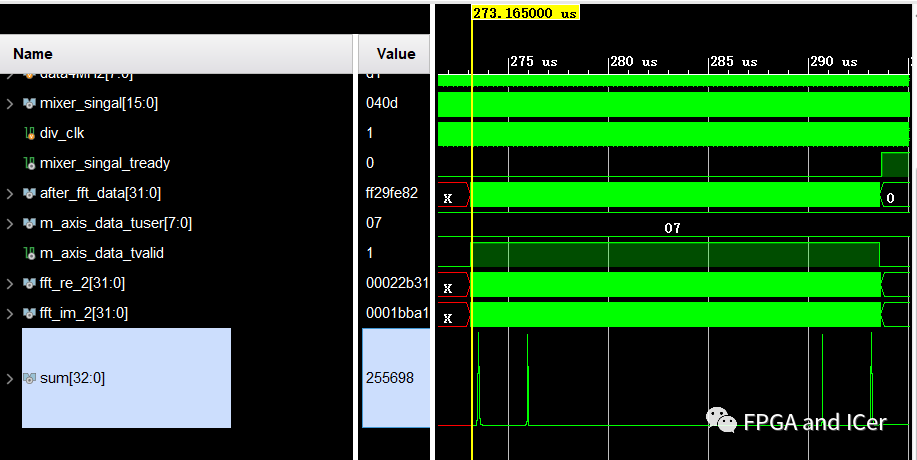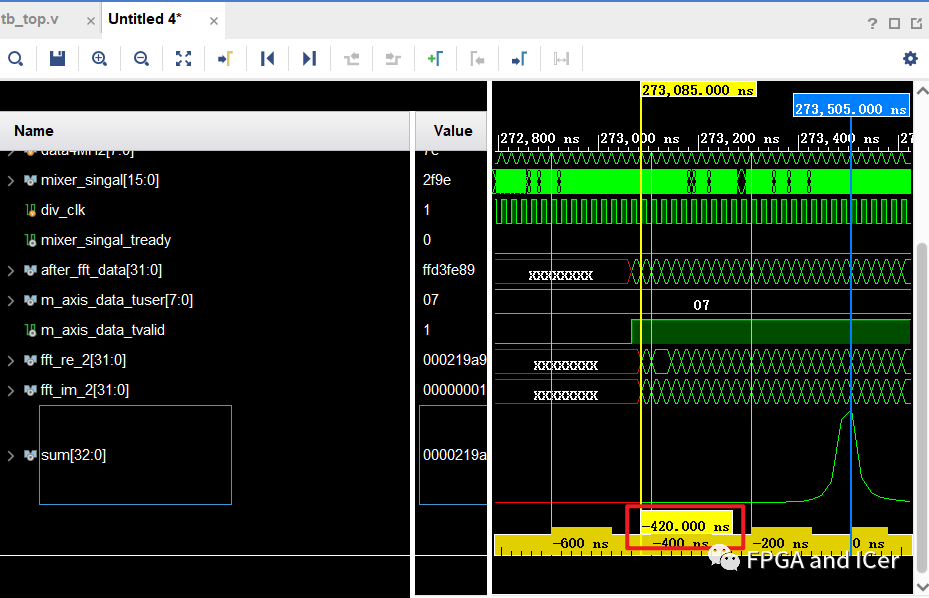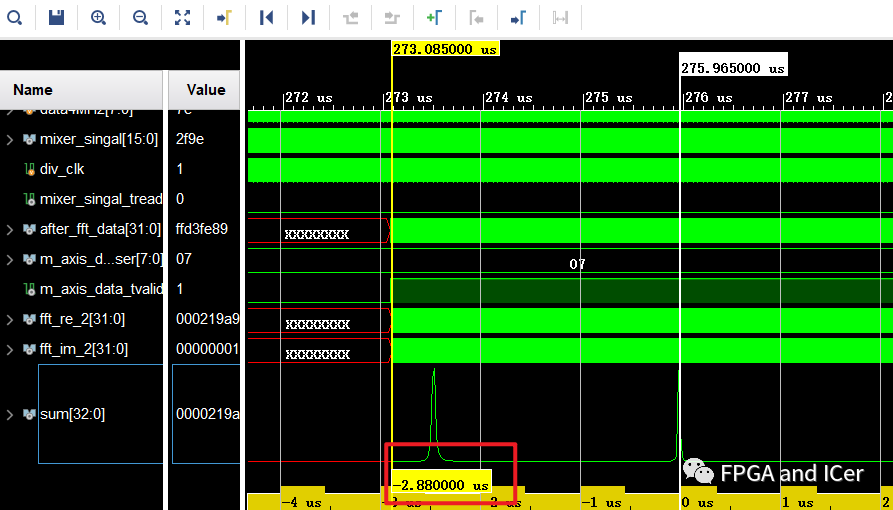×
20